Register for Maths, Science, English, Reasoning Olympiad Exams Register here | Book Free Demo for Live Olympiad Classes here| Check Olympiad Exam Dates here | Buy Practice Papers for IMO, IOM, HEO, IOEL etc here | Login here to participate in all India free mock test on every Saturday

Work and Energy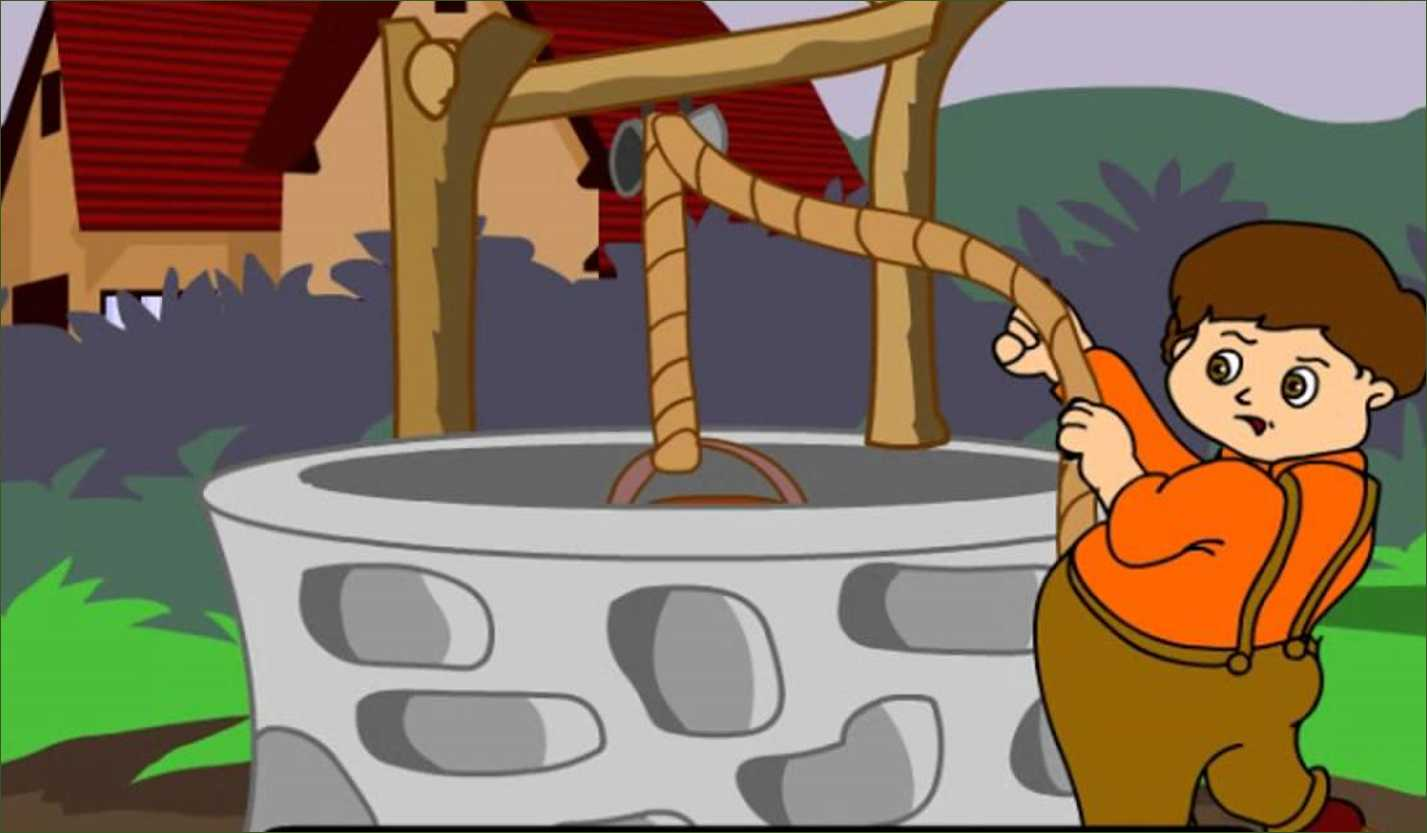Every living being on planet earth needs food to sustain their life. Food is the source of energy for any living being and helps the living organism work and survive on the earth. Animals always need to do physical activities to survive and these work and energy help them to go through this process in an easy manner.

Scientific Conception of Work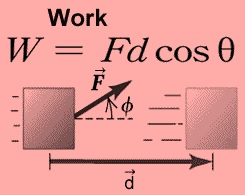Push a pebble lying on a surface. The pebble moves through a distance. You exerted a force on the pebble and the pebble got displaced. In this situation, work is done.

A girl pulls a trolley and the trolley moves through a distance. The girl has exerted a force on the trolley and it is displaced. Therefore, work is done.

Lift a book through a height. To do this you must apply a force. The book rises. There is a force applied to the book and the book has moved. Hence, work is done.

A closer look at the above situations reveals that two conditions need to be satisfied for work to be done:

(i) A force should act on an object

(ii) The object must be displaced.

If anyone of the above conditions does not exist, work is not done. This is the way we view work in science.

Work Done By a Constant Force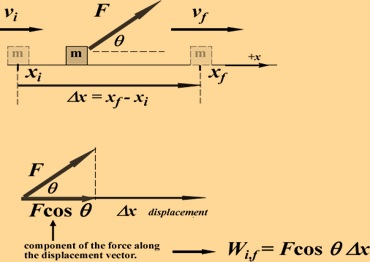Thus, work done by a force acting on an object is equal to the magnitude of the force multiplied by the distance moved in the direction of the force. Work has only magnitude and no direction.

Thus 1 J is the amount of work done on an object when a force of 1 N displaces it by 1 m along the line of action of the force.

Energy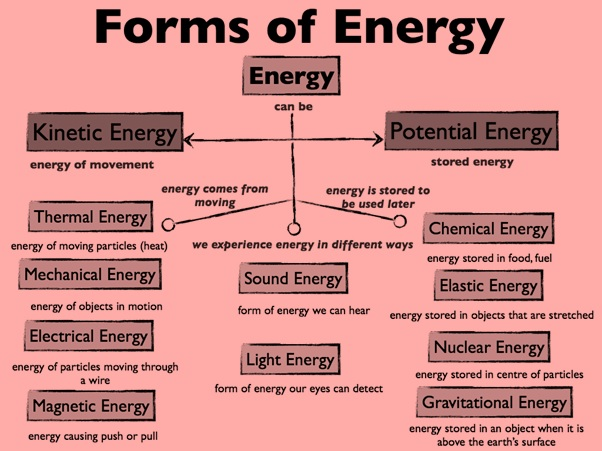Life is impossible without energy. The demand for energy is ever increasing. The Sun is the biggest natural source of energy to us. Many of our energy sources are derived from the Sun. We can also get energy from the nuclei of atoms, the interior of the earth, and the tides.

The energy possessed by an object is thus measured in terms of its capacity of doing work. The unit of energy is, therefore, the same as that of work, that is, joule(J). 1 J is the energy required to do 1 joule of work. Sometimes a larger unit of energy called kilojoule (kJ) is used. 1 kJ equals 1000 J.

Kinetic Energy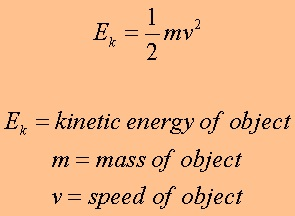By definition, we say that the kinetic energy of a body moving with a certain velocity is equal to the work done on it to make it acquire that velocity. A falling coconut, a speeding car, a rolling stone, a flying aircraft, flowing water, blowing wind, a running athlete etc. possess kinetic energy. In short, kinetic energy is the energy possessed by an object due to its motion. The kinetic energy of an object increases with its speed.

Potential Energy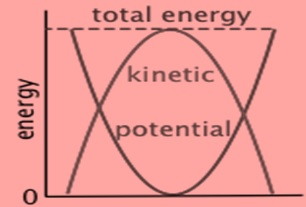The energy gets stored due to the work is done on the object. The energy transferred to an object is stored as potential energy if it is not used to cause a change in the velocity or speed of the object. You transfer energy when you stretch a rubber band. The energy transferred to the band is its potential energy. You do work while winding the key of a toy car. The energy transferred to the spring inside is stored as potential energy. The potential energy possessed by the object is the energy present in it by virtue of its position or configuration.

A Rate of Doing Work: Power

The speed with which these vehicles change energy or do work is a basis for their classification. Power measures the speed of work done, that is, how fast or slow work is done. Power is defined as the rate of doing work or the rate of transfer of energy. If an agent does a work W in time t, then power is given by:

W=p/t

The unit of power is watt having the symbol W. 1 watt is the power of an agent, which does work at the rate of 1 joule per second.

Recap

• Work done on an object is defined as the magnitude of the force multiplied by the distance moved by the object in the direction of the applied force. The unit of work is joule: 1 joule = 1-newton × 1 meter.
• Work done on an object by a force would be zero if the displacement of the object is zero.
• An object having the capability to do work is said to possess energy. Energy has the same unit as that of work.
• An object in motion possesses what is known as the kinetic energy of the object. An object of mass, m moving with velocity v has a kinetic energy of (1/2)XmXvXv.
• The energy possessed by a body due to its change in position or shape is called the potential energy. The gravitational potential energy of an object of mass, m raised through a height, h from the earth’s surface is given by mg h.
• According to the law of conservation of energy, energy can only be transformed from one form to another; it can neither be created nor destroyed. The total energy before and after the transformation always remains constant.
• Energy exists in nature in several forms such as kinetic energy, potential energy, heat energy, chemical energy etc. The sum of the kinetic and potential energies of an object is called it's mechanical energy.
• Power is defined as the rate of doing work. The SI unit of power is watt. 1 W = 1 J/s.
• The energy used in one hour at the rate of 1kW is called 1 kWh.Quiz for Work and Energy

 Q.1 What is the relation between 1 J and 1 calorie? a) 1 J = 4.18 Cal b) 1 J = 2 cal c) 1 cal = 4.18 J d) 1 cal = 6 J
 Q.2 Work and energy are related by ___________________. a) Heat transfer coefficient b) Heat absorption coefficient c) Stefan' s coefficient d) Mechanical equivalent of heat
 Q.3 What is the work to be done to increase the velocity of a car from 8.33 m/s to 16.67 m/s if the mass of the car is 1500 kg? a) 150 kJ b) 156.38 kJ c) 160 kJ d) 188 kJ
 Q.4 Which of them can be a form of kinetic energy? a) Energy possessed by a bullet b) Energy possessed by a train c) Energy possessed by a car d) All of them are correct
 Q.5 A car weighing 20kN has speed of 20 m/s. Find its kinetic energy? a) 2400 kJ b) 240 kJ c) 2400 J d) 240 J
 Q.6 How much is the work done in pulling up a block of wood weighing 2KN for a length on a smooth plane inclined at an angle of 30o with the horizontal? a) 1.732 KJ b) 10 KJ c) 100 KJ d) 17.32 KJ
 Q.7 A force of 7N, making an angle θ with the horizontal, acting on an object displaces it by 0.5m along the horizontal direction. If the object gains K.E. of 2J, what is the horizontal component of the force? a) 1 N b) 2 N c) 4N d) 14 N
 Q.8 A 60kg with 10 kg load on his head climbs 25 steps of 0.20m height each. What is the work done in climbing? (g=10m/s2) a) 3500 J b) 5 J c) 350 J d) 100 J
 Q.9 The mass of a car is 1000 kg. How much work is required to be done on it to make it move with a speed of 36 km/h? a) 2.5 x 104 J b) 5 x 103 J c) 500 J d) 5 x 104 J
 Q.10 A 8kg mass moves along x-axis. Its acceleration as a function of its position is shown in the figure. What is the total work done on the mass by the force as the mass moves from x=0 to x=6cm?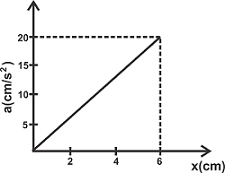a) 4.8 x 10-3 J b) 48 x 10-3 J c) 98 x 10-3 J d) 9.8 x 10-3 J